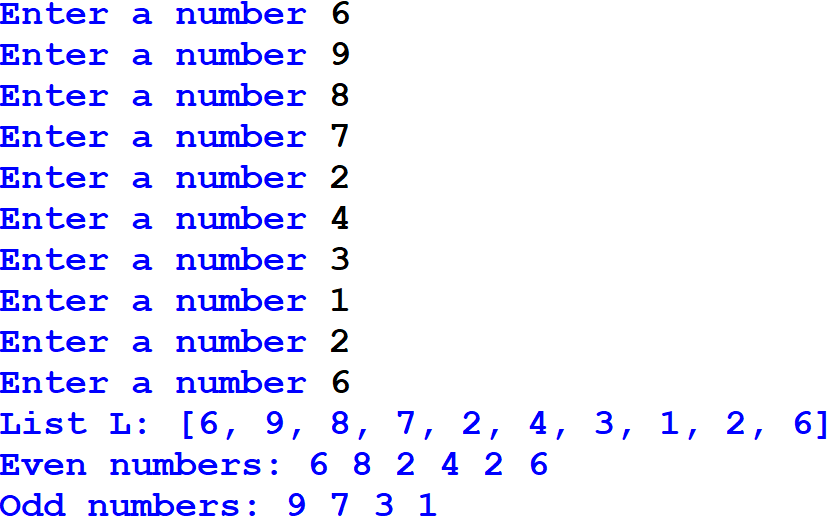## Python program that asks the user to enter 5 integers and stores them in list L. Then, a function is created that takes list L and prints list L and all even numbers and odd numbers in the list L.

Write a program that asks the user to enter 5 integers and stores them in list L. Create a function that takes list L and prints list L and all even numbers and odd numbers in the list L.

Sample output:L = []
for i in range (5):
x = int(input("Enter a number "))
L.append(x)
print("List L: ",L)
def num(L):
even = []
odd = []
for x in L:
if x % 2 == 0:
even.append(x)
elif x % 2 != 0:
odd.append(x)
print("Even numbers: ",even)
print("Odd numbers: ",odd)
num(L)